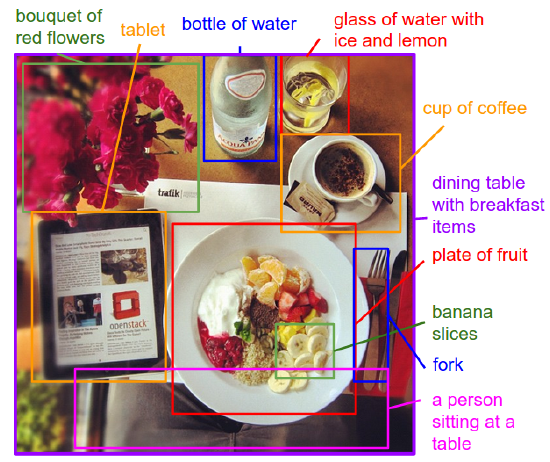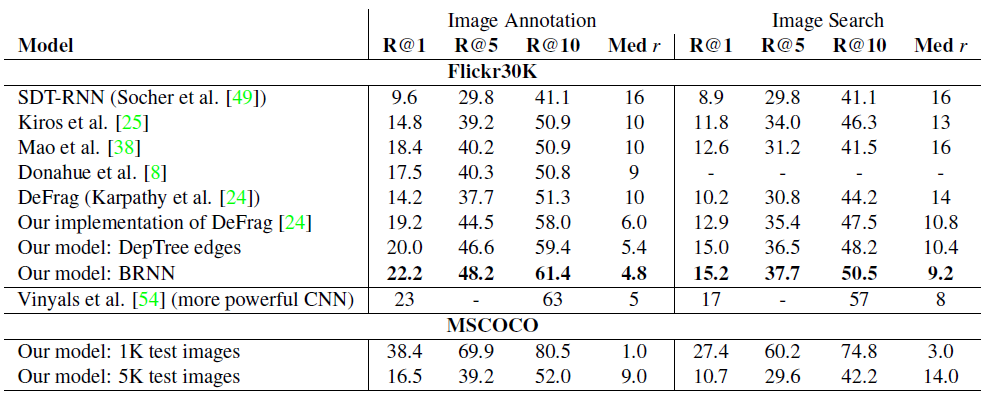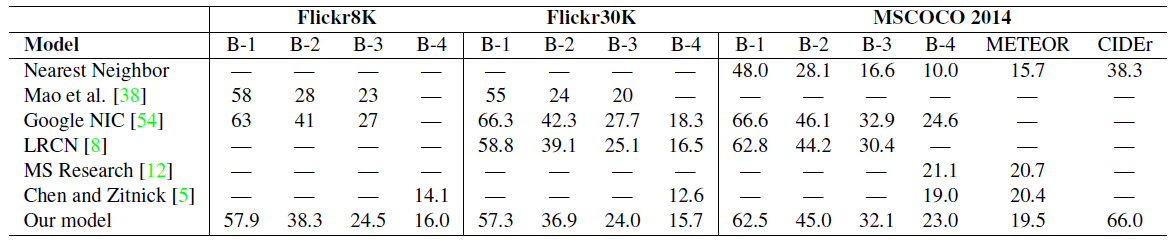# Review — Deep Visual-Semantic Alignments for Generating Image Descriptions

## CNN for Image, Bidirectional RNN for Senstences, Generate Descriptions over Images RegionsMotivation/Concept Figure: The proposed model treats language as a rich label space and generates descriptions of image regions

In this story, Deep Visual-Semantic Alignments for Generating Image Descriptions, by Stanford University, is reviewed. This is a paper by Prof. Li Fei-Fei, In this paper:

• A Convolutional Neural Network (CNN) is used over image regions, and a bidirectional Recurrent Neural Network is used over sentences, and a structured objective that aligns the two modalities through a multimodal embedding, and generate novel descriptions of image regions.

This is a paper in 2015 CVPR and 2017 TPAMI with over 4600 citations where TPAMI has high impact factor of 16.389. (Sik-Ho Tsang @ Medium)

# Outline

1. R-CNN+BRNN Model Framework for Regions
2. Multimodal RNN Model Framework for Full Image
3. Experimental Results

# 1. R-CNN+RNN Model Framework for Regions

• The framework consists of R-CNN object detection network for extracting 20-region image representation from one single image, bidirectional RNN for extracting word representation.
• And finally, an image-sentence score mechanism to output the most relevant word.

## 1.1. Image Representation

• R-CNN is used to detect objects in every image.

The top 19 detected locations in addition to the whole image (20 in total) are used to compute the representations based on the pixels Ib inside each bounding box:

• where CNN(Ib) transforms the pixels inside bounding box Ib into 4096-dimensional activations of the fully connected layer immediately before the classifier.
• The CNN parameters θc contain approximately 60 million parameters.
• The matrix Wm has dimensions h×4096, where h is the size of the multimodal embedding space (h ranges from 1000-1600 in our experiments).

Every image is thus represented as a set of h-dimensional vectors.

## 1.2. Sentence Representation

A Bidirectional Recurrent Neural Network (BRNN) takes a sequence of N words (encoded in a 1-of-k representation) and transforms each one into an h-dimensional vector.

• However, the representation of each word is enriched by a variably-sized context around that word. Using the index t=1…N to denote the position of a word in a sentence, the precise form of the BRNN is as follows:
• Here, It is an indicator column vector.
• The weights Ww specify a word embedding matrix that is initialized with 300-dimensional word2vec weights and keep fixed due to overfitting concerns. (word2vec is proposed in a 2013 NeurIPS paper to convert words into vectors, with over 29000 citations.)
• BRNN consists of two independent streams of processing, one moving left to right (hft) and the other right to left (hbt).
• Technically, every st is a function of all words in the entire sentence.
• A typical size of the hidden representation here ranges between 300–600 dimensions.
• f is ReLU.

## 1.3. Alignment Objective

• The strategy is to formulate an image-sentence score as a function of the individual region-word scores.
• Intuitively, a sentence-image pair should have a high matching score if its words have a confident support in the image.
• The model of Karpathy et a.  interprets the dot product of vTi and st between the i-th region and t-th word as a measure of similarity and use it to define the score between image k and sentence l as:
• Here, gk is the set of image fragments in image k and gl is the set of sentence fragments in sentence l.
• The following reformulation simplifies the model:
• Here, every word st aligns to the single best image region.
• Assuming that k = l denotes a corresponding image and sentence pair, the final max-margin, structured loss remains:

This objective encourages aligned image-sentences pairs to have a higher score than misaligned pairs, by a margin.

## 1.4. Decoding Text Segment Alignments to Images

• The dot product quantity vTi st can be interpreted as the unnormalized log probability of the t-th word describing any of the bounding boxes in the image. However, the ultimate interest is to generate snippets of text instead of single words.
• The true alignments are treated as latent variables in a Markov Random Field (MRF).
• Concretely, given a sentence with N words and an image with M bounding boxes, the latent alignment variables aj ∈ {1…M} are introduced for j=1…N and an MRF is formulated in a chain structure along the sentence as follows:
• Here, β is a hyperparameter that controls the affinity towards longer word phrases.
• The first term is the unary term, the highest score for each word.
• The second term is the interaction potential, adjacent words that are encouraged to be assigned to the same bounding box.
• The energy is minimized to find the best alignments a using dynamic programming.

In brief, the output of this process is a set of image regions annotated with segments of text. MRF is used as non-deep-learning post-processing stage to generate short snippets.

MRF or CRF has also been used in semantic segmentation in early development such CRF-RNN and DeepLab. Indeed, deep neural network can be used to replace MRF/CRF.

# 2. Multimodal RNN Model Framework for Full Image

• In this full-image model, the RNN takes a word, the context from previous time steps and defines a distribution over the next word in the sentence.
• The RNN is conditioned on the image information at the first time step. START and END are special tokens.
• The cost function is to maximize the log probability assigned to the target labels.

# 3. Experimental Results

• Flickr8K, Flickr30K and MSCOCO datasets are evaluated.

## 3.1. RegionsImage-Sentence ranking experiment results. R@K is Recall@K (high is good). Med r is the median rank (low is good).
• Compared to other work that uses AlexNets, the proposed full model (BRNN) shows consistent improvement.
• BRNN is taking advantage of contexts longer than two words. Furthermore, it does not rely on extracting a Dependency Tree and instead uses the raw words directly.
• For every test image above, the most relevant word is retrieve and the highest-scoring region before MRF smoothing is shown.
• The model discovers interpretable visual-semantic correspondences, even for small or relatively rare objects such as an “accordion”.

## 3.2. Full ImageEvaluation of full image predictions on 1,000 test images. B-n is BLEU score that uses up to n-grams.
• The Multimodal RNN confidently outperforms this retrieval method.
• Additionally, the RNN takes only a fraction of a second to evaluate per image.
• The first prediction “man in black shirt is playing a guitar” does not appear in the training set. However, there are 20 occurrences of “man in black shirt” and 60 occurrences of “is paying guitar”, which the model may have composed to describe the first image.
• In general, a relatively large portion of generated sentences (60% with beam size 7) can be found in the training data.
• This fraction decreases with lower beam size; For instance, with beam size 1 this falls to 25%, but the performance also deteriorates (e.g. from 0.66 to 0.61 CIDEr).
• The region-level multimodal RNN is used to generate text (shown on the right of each image) for some of the bounding boxes in each image.
• The region RNN model produces descriptions most consistent with the collected data.
• Note that the full-frame model was trained only on full images, so feeding it smaller image regions deteriorates its performance. However, its sentences are also longer than the region model sentences, which likely negatively impacts the BLEU score.

## References

[2015 CVPR] [R-CNN+BRNN]
Deep Visual-Semantic Alignments for Generating Image Descriptions
[2017 TPAMI] [R-CNN+BRNN]
Deep Visual-Semantic Alignments for Generating Image Descriptions

## Natural Language Processing (NLP)

2014 [Seq2Seq] [RNN Encoder-Decoder] 2015 [m-RNN] [R-CNN+BRNN]

--

--